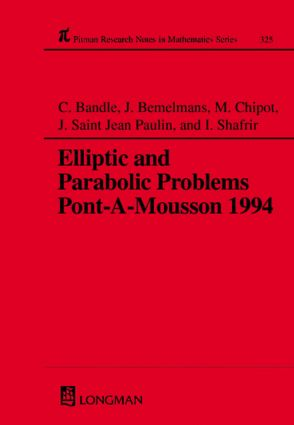# Elliptic and Parabolic Problems

## Pont-A-Mousson 1994, Volume 325, 1st Edition

Chapman and Hall/CRC

272 pages

Hardback: 9780582239616
pub: 1995-04-26
\$185.00
x

FREE Standard Shipping!

### Description

This Research Note presents some recent advances in various important domains of partial differential

equations and applied mathematics including equations and systems of elliptic and parabolic type and various applications in physics,

mechanics and engineering.

These topics are now part of various areas of science and have

experienced tremendous development during the last decades.

-------------------------------------

Preface

Calderon-Zygmund inequalities, Riesz potentials and Riesz transforms in weighted Sobolev spaces, C Amrouche,

V Girault and J Giroire

Nonuniqueness results forquasilinear elliptic systems, N Andre

The support shrinking in solutions of parabolic equations with non

homogeneous absorption terms, S N Antontsev, J I Diaz and S I Shmarev

Representation and approximation of solutions of initial value problems for differential equations in Hilbert space based on the Cayley transform, D Z Arov, I P Gavrilyuk

and V L Makarov

A unified formulation for the boundary conditions in some

convection diffusion problem, J Carrillo and A Alonso

An existence theorem for an unbounded dam with leaky boundary

conditions, M Chipot and A Lyaghfouri

Local weak solutions for a multivalued evolution equation, M Choulli and R Deville

Nonlinear parabolic problems modelling transition dynamics with

memory, P Colli and M Grasselli

On a multi dimensional moving boundary problem, J Escher and G

Simonett

Cauchy problem for equations of nonlinear heat conductibility with

convection in classes of growing functions, A L Gladkov

Large time behaviour of non isothermal models for phase separation, A Ito, N Kenmochi and M Niezgodka

On convergence of the solutions of inverse problems for parabolic

equations with weakly converging coefficients, V L Kamynin

On the Airy equation with a coefficient depending on t, J

Popiolek

Radial symmetry by moving planes for semilinear elliptic BVPs on annuli and other non convex domains, W

Reichel

Large time behavior of the solution to a phase change problem with

constraint, N Sato, J Shirohzu and N Kenmochi

Entire positive solutions for semi linear elliptic functions, A Simon

On quasilinear parabolic higher order equations with Holder

continuous solutions, I V Skrypnik

Existence and uniqueness results for degenerate elliptic integro

differential problems, M A Vivaldi

Application of the Leray-Schauder degree to investigation of

travelling wave solutions of parabolic equations, A I Volpert and

V A Volpert

Degenerate elliptic models for perfectly plastic flows, H F

Weinberger

Some recent results on the large time behavior for scalar parabolic

conservation laws, E Zuazua